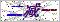订购服务热线： 400-053-8065首页 - 产品中心 - 工业控制传感器/变送器 - 温度传感器/变送器（热电偶、热电阻） - 温度探头、pt100温度传感器
 产品目录工业控制传感器/变送器产品中心点击放大
 温度探头、pt100温度传感器
 产品型号： 产品报价：产品特点： 温度探头、pt100温度传感器、温度传感器、JCJ100TL全螺纹温度传感器是指测温探头部分全部采用螺纹结构封装，内部填充绝缘导热材料密封而成。通过调节螺纹部分长度来测量（以螺纹方式固定的）物体表面温度，也可测量轴承和轴瓦表面温度，一般螺纹部分长度较短。如果要求传感器探头较长，则采用螺纹和保护管组合在一起测温。
 温度探头、pt100温度传感器的详细资料：JCJ100TL 全螺纹固定温度传感器选型 铂热电阻类型 P口 0=Pt100     1=Pt1000       5=Pt500     6=Pt10　                9=指定 温度范围(℃) T口 0=0～80  1=-50～100  2=-50～150  3=-50～200    4=-50～300      9=指定 传感器精度 口 A=A级      B=B级 保护管材质 C口 1=SUS321   4=SUS304    6=SUS316       9=指定 螺纹规格 M口 1=M8×1  2=M8×1.25  3=M10×1  4=M10×1.5  5=M12×1  6=M12×1.5   7=M16×1.5   9=指定 螺纹长度(mm) G口 40=40   50=50   60=60   80=80  100=100                         9=指定 引线长度(mm) L口 1=100   2=200   3=300   5=500  10=1000  30=3000  50=5000       9=指定 引出线采用线制 X口 2=两线制  3=三线制   4=四线制 引线材质 口 1=聚氯乙烯PVC（-20～80℃）   分有屏蔽层和无屏蔽层两种2=硅橡胶     （-60～150℃）  无屏蔽层3=四氟绕包   （-50～250℃）  有屏蔽层    4=云母绕包   （-80～500℃）  无屏蔽层                    9=指定 是否配屏蔽层 口 0=无屏蔽层（默认）      1=有屏蔽层 导线末端要求 口 0=无   1=镀锡   2=U型端子   3=针式端子                   9=指定 特殊要求 Q口 0=无   9=指定 注意事项：1、选用云母绕包线时，螺纹长度≥50mm，配其它线时，螺纹长度≥40mm，特殊情况定制。                    2、选用云母绕包线时，螺纹部分直径≥16mm ，配其它线缆时，螺纹部分直径≥8mm ，特殊情况定制。

 JCJ100TL 全螺纹固定数字温度传感器选型 传感器类型 D 数字温度传感器DS18B20    （有关数字DS18B20介绍请见第4页相关内容） 温度范围(℃) T口 0=－20～80    1=-55～125 保护管材质 C口 1=SUS321   4=SUS304    6=SUS316       9=指定 螺纹规格 M口 1=M10×1  2=M10×1.5   3=M12×1  4=M12×1.5   5=M16×1.5   9=用户指定 螺纹长度(mm) G口 40=40   50=50　 60=60   80=80  100=100  150=150     9=指定 引线长度(mm) L口 1=100   2=200   3=300   5=500  10=1000  30=3000  50=5000  9=指定 引线材质 口 1=聚氯乙烯PVC（-20～80℃）   2=硅橡胶（-60～150℃）无屏蔽层 是否配屏蔽层 口 0=无屏蔽层（默认）    1=有屏蔽层 导线末端要求 口 0=无   1=镀锡    2=U型端子   3=针式端子   9=指定 特殊要求 Q口 0=无      9=指定

 如果你对温度探头、pt100温度传感器感兴趣，想了解更详细的产品信息，填写下表直接与厂家联系：

### 留言框

• #### 验证码：请输入计算结果（填写阿拉伯数字），如：三加四=7无锡斯洛森测控技术发展有限公司(www.wxckyb.com) 版权所有 热卖产品：露点仪,露点测量仪,在线露点传感器,钢水测温仪,铝材测温仪,在线红外测温仪 总访问量：160762 地址：无锡市崇安区江海东路588号21栋604室 邮编：224200 电话：86-0510-66810836 传真：86-0510-82300399 手机：15852523519 联系人：朱小明 邮箱：2818992521@qq.com GoogleSitemap 技术支持：仪表网 管理登陆 ICP备：苏ICP备14044566号-1